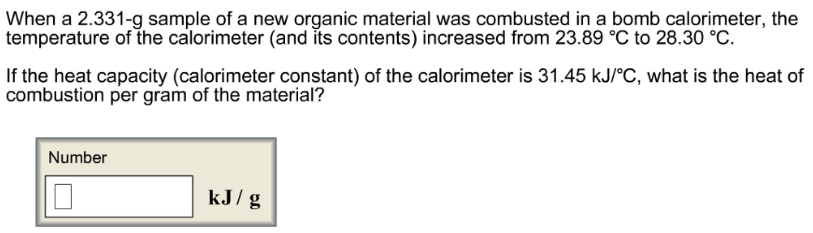# Problem: When a 2.331-g sample of a new organic material was combusted in a bomb calorimeter, the temperature of the calorimeter (and its contents) increased from 23.89  C to 28.30 C. If the heat capacity (calorimeter constant) of the calorimeter is 31.45 kJ/ C, what is the heat of combustion per gram of the material?

###### FREE Expert Solution
86% (230 ratings)###### Problem Details

When a 2.331-g sample of a new organic material was combusted in a bomb calorimeter, the temperature of the calorimeter (and its contents) increased from 23.89  C to 28.30 C. If the heat capacity (calorimeter constant) of the calorimeter is 31.45 kJ/ C, what is the heat of combustion per gram of the material?